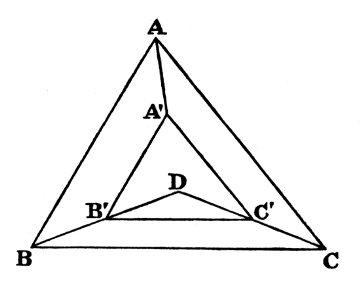#Pillow-Problems: Problem #26

Math Lair Home > Source Material > Pillow-Problems > Problem #26

## Problem:

26.

Within a given Triangle place a similar Triangle, whose area shall have to its are a given ratio less than unity, whose sides shall be parallel to its sides, and whose vertices shall be equidistant from its vertices.

[4/89

## Solution:

26.

#### (Analysis.)

Let ABC be the given Triangle, and ABC′ the required one; and let the ratio, which BC′ has to BC, be ‘k’; so that k is less than 1.

Since BB′ = CC′, and that BC, BC′, are parallel, it may easily be proved, by dropping perpendiculars from B′, C′, upon BC, which must necessarily be equal, that ∠s BBC, CCB, are equal.

Similarly, ∠s AAC, CCA are equal; and so are ∠s AAB, BBA.Call ∠BBC ‘θ’; then ∠CCB = θ

∴ ∠CCA = C − θ = ∠ AAC;

∴ ∠AAB = A − (C − θ) = ∠BBA.

Now ∠s BBC, BBA, together = B;

∴ θ + A − (C − θ) = B;

∴ 2θ = B + CA = 180° − 2A;

∴ θ = 90° − A.

Hence, if BB′, CC′, be produced to meet at D, Triangle DBC will be isosceles, with a vertical ∠ equal to 2A.

Now, if a Circle be drawn about ABC, and its centre joined to B and C, the Triangle, so formed, will fulfil the same conditions;

hence the centre of this Circle will be D;

hence the construction.

#### (Synthesis.)

Bisect the sides, and draw perpendiculars, meeting at D. Join D to the vertices B, C. From DB cut off DB′ = k · DB. From B′ draw BC′ parallel to BC.

Then BC′ is easily proved equal to k · BC.

And if, from B&prime, C′, parallels to AB, AC, be drawn, it may easily be proved that they meet on DA, and that they are respectively equal to k · AB, k · AC.

Q.E.F.